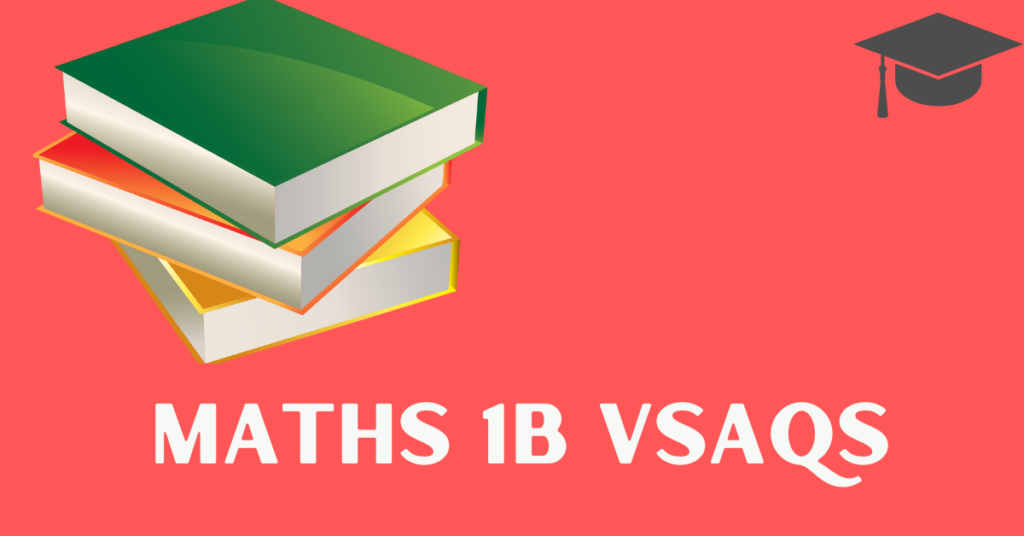# Inter Maths 1B – Inter 2marks Q & S || V.S.A.Q’S||

TS VI to IX Math’s Material

TS X Math’s Material

TS Inter Math’s & Accountancy Material

CBSE & ICSE Material

Quantitative Aptitude

Play Quiz Game and Test Your Score

Polytechnic Mathematics

Inter Maths 1A  V.S.A.Q.’S

Inter Maths 1B V.S.A.Q’S

## Inter Maths 1B

This content was designed by the ‘Basics in Maths’ team. These notes to do help the intermediate First-year Maths students.

website has been given material for mathematics

First-year Maths students.

Inter Maths – 1B  2 marks questions and solutions are very useful in IPE examinations.

### Inter Maths 1B – Inter 2marks Q & S

TS VI to IX Math’s Material

TS X Math’s Material

TS Inter Math’s & Accountancy Material

CBSE & ICSE Material

Quantitative Aptitude

Play Quiz Game and Test Your Score

Polytechnic Mathematics

Inter Maths 1A  V.S.A.Q.’S

Inter Maths 1B V.S.A.Q’S

### Visit my Youtube Channel:Click on Below Logo